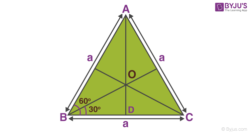Know more about the Repeaters Batch Know more about the Repeaters Batch

# Centre of Mass of Equilateral Triangle

In geometry, the equilateral triangle is a triangle in which all the three sides are equal. All the internal angles of the equilateral triangle are also equal. The angles are equal to 600.

Test your Knowledge on Equilateral Triangle
The centre of mass is the point in the body or the system of bodies at which the whole mass of the body is considered to be concentrated. We must also know that the centroid is the geometrical centre of the object. In other words, the exact centre of the object is also known as the centroid of that object. In an equilateral triangle, the centroid and centre of mass are the same. The centroid or centre of mass of an equilateral triangle is the point at which its medians meet.

## How to Find Centre of Mass of Equilateral TriangleThe centre of mass can be calculated by following these steps

Step 1: Find the midpoint of all the three sides of the triangle.

Step 2: Draw a perpendicular from midpoint to the opposite vertex. This perpendicular line is called the median.

Step 3: These three medians meet at a point. This point of intersection of the medians is the centre of mass of the equilateral triangle.

The centre of mass of the equilateral triangle is at a distance of H/3 from the centre of the base of the triangle. H is the height of the triangle. The centroid or the centre of mass divides the median in 2:1 ratio.

## Calculation of Distance of the Centre of Mass from the Vertex

Let a be the length of the sides. The internal angle of the equilateral triangle is 600.

Using Pythagoras theorem,

Median of the equilateral triangle divides the median by the ratio 2:1

Therefore, OD = (a√3)/6

In triangle OBD

Sin 300 = OD/OB

Sin 300 = ((a√3)/6)/OB

OB = (a√3)/3

For the triangle of side a, the distance from the centre of mass to the vertex is (a√3)/3.